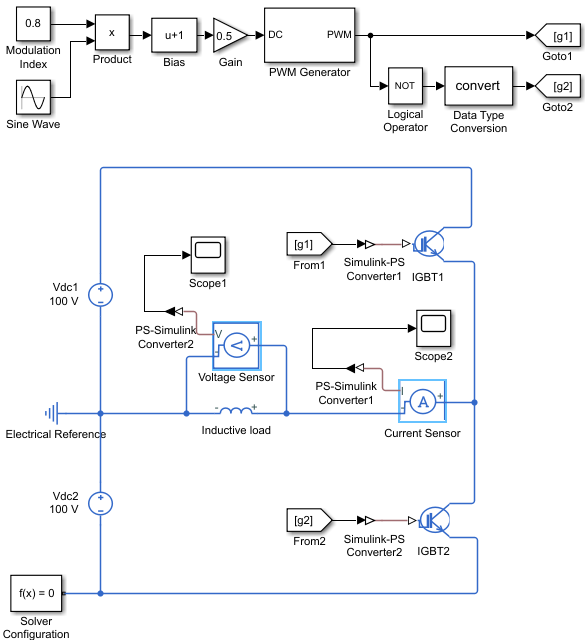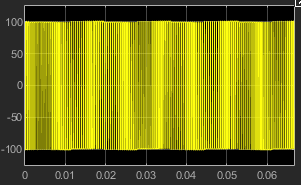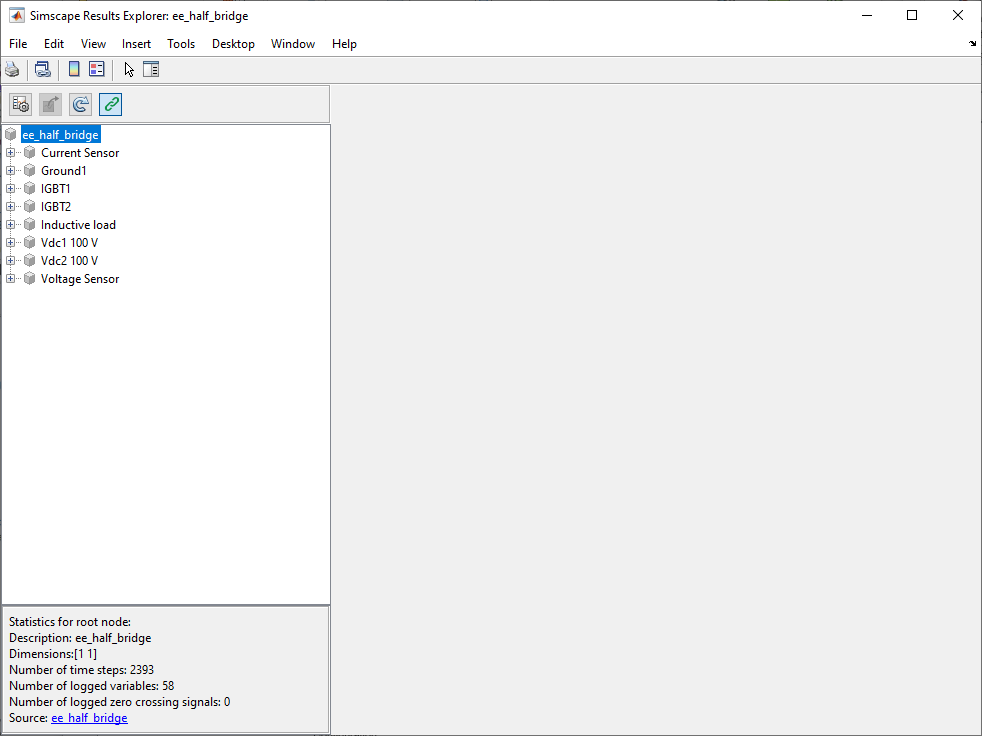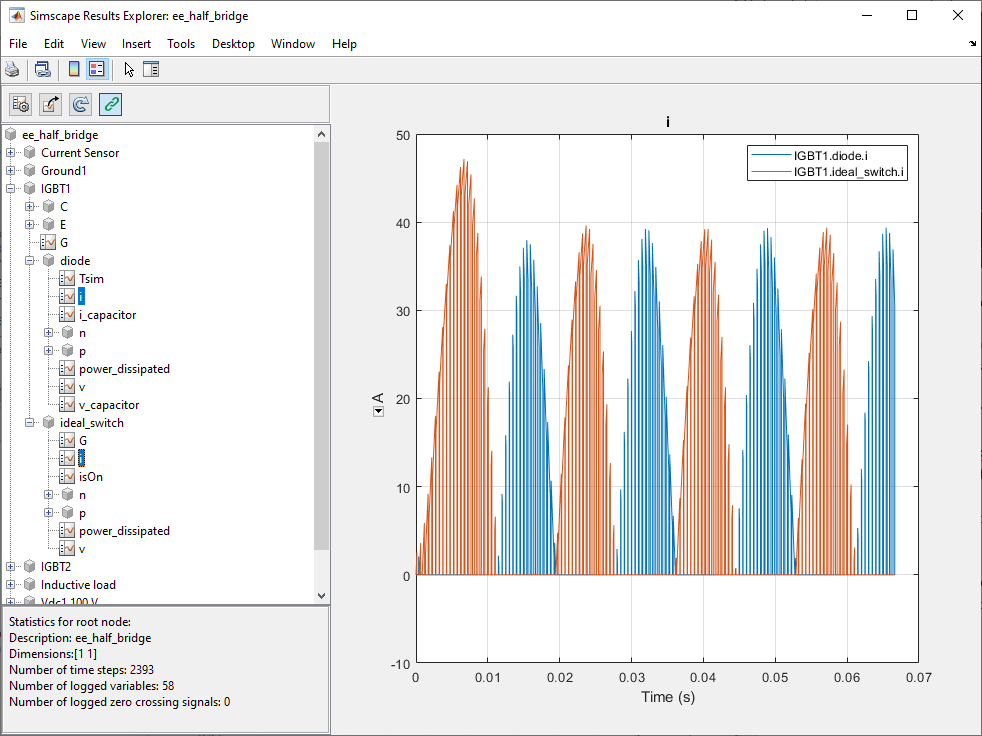## Build and Simulate a Single-Phase Half-Bridge Inverter with Ideal Switches

In this example, you build and analyze a single-phase, half-bridge inverter that you control by using sinusoidal pulse-width modulation (SPWM).

To see the completed version of the model you create in this example, at the MATLAB command prompt, enter `ee_half_bridge`.

### Build the Model

The single-phase, half-bridge inverter in this example consists of a power circuit and a control system. First, create both parts of the model by adding and connecting the blocks.

#### Build the Power Circuit

The power circuit comprises a DC link, two semiconductor switches with their anti-parallel diodes, and an inductive load.

To build the power circuit:

1. Create a new model.

2. Add the blocks in this table. The Library Path column specifies the hierarchical path to each block.

Block

Purpose

Library Path

Quantity

DC Voltage SourceProvide a DC linkSimscape > Foundation Library > Electrical > Electrical Sources

2

IGBT (Ideal, Switching)Model two semiconductor switches with their anti-parallel diodes and build the half-bridge

Simscape > Electrical > Semiconductor & Converters

2

InductorModel an inductive loadSimscape > Foundation Library > Electrical > Electrical Elements

1

Electrical ReferenceProvide the ground connection for the electrical conserving portsSimscape > Foundation Library > Electrical > Electrical Elements

1

Voltage SensorMeasure the voltage across the loadSimscape > Foundation Library > Electrical > Electrical Sensors

1

Current SensorMeasure the current that flows through the loadSimscape > Foundation Library > Electrical > Electrical Sensors

1

Solver ConfigurationDefine the solver settings that apply to all physical modeling blocksSimscape > Utilities

1

Simulink-PS ConverterConvert an output physical signal to a Simulink signalSimscape > Utilities

2

PS-Simulink Converter Convert a Simulink input signal into a physical signalSimscape > Utilities

2

ScopeDisplay the load voltage and the current waveSimulink > Commonly Used Blocks

2

Note

You can use the Simscape™ function `ssc_new` with a domain type of `electrical` to create a Simscape model that contains these blocks:

• Scope

• Solver Configuration

• Electrical Reference

3. Rename and connect the blocks as shown in the diagram.#### Build the Control System

The control system generates two pulse-width modulated (PWM) gate signals that control the switching behavior of the two IGBT blocks. If the gate signal value is equal to `1`, the IGBT (Ideal, Switching) block turns on. If the gate signal value is equal to `0`, the block turns off.

To build the control system:

1. Add the blocks in this table to the model. The Library Path column specifies the hierarchical path to each block.

Block

Purpose

Library Path

Quantity

Sine WaveGenerate a sinusoidal signal that represents a unit sinusoidal modulation waveSimulink > Sources1
ConstantDefine the sinusoidal pulse-width modulation indexSimulink > Sources1
ProductObtain the sinusoidal modulation wave by multiplying the unit sinusoidal wave by the modulation indexSimulink > Math Operations

1

BiasPerform calculation to obtain the duty cycle signalSimulink > Math Operations

1

GainSimulink > Math Operations

1

PWM GeneratorGenerate the PWM gate signal for the `IGBT1` blockSimscape > Electrical > Control > Pulse Width Modulation

1

Logical OperatorObtain the complementary PWM gate signal for the `IGBT2` blockSimulink > Logic and Bit Operations

1

Data Type ConversionConvert the input Boolean signal to double signalSimulink > Signal Attributes

1

Goto Pass the input PWM gate signal to the corresponding From blockSimulink > Signal Routing

2

FromReceive a PWM gate signal from the corresponding Goto block and pass the signal as an outputSimulink > Signal Routing

2

2. Rename and connect the blocks as shown in the diagram.### Specify Model Parameters

Specify these parameters to represent the behavior of the system components:

#### Model Setup Parameters

These blocks specify model information that is not specific to a particular block:

• Solver Configuration

• Electrical Reference

As with Simscape models, you must include a Solver Configuration block in each topologically distinct physical network. This example has a single physical network, so use one Solver Configuration block with the default parameter values.

You must include an Electrical Reference block in each Simscape Electrical™ network. This block does not have any parameters.

#### Power Circuit Parameters

Specify these parameters to represent the behavior of the power circuit components:

1. Set the Constant voltage parameter of the two DC Voltage Source blocks to `100`.

2. Set the parameters of the two IGBT (Ideal, Switching) blocks:

• Main settings

• Forward voltage, Vf`0.8`

• On-state resistance`0.001`

• Off-state conductance`1e-5`

• Threshold voltage, Vth`0.5`. With this value, the IGBT switches on when the value at the input port is equal to `1` and it switches off when the value at the input port is equal to `0`.

• Integral Diode settings

• Integral protection diode```Protection diode with no dynamics```

• Forward voltage`0.8`

• On resistance`0.001`

• Off conductance`1e-5`

3. Set the parameters of the Inductor block:

• Inductance`0.005`

• Series resistance`1`

• Parallel conductance`0`

#### Control System Parameters

Specify the following parameters to get the PWM gate signals for the `IGBT1` and `IGBT2` from a sinusoidal modulation wave:

1. In the Constant block, set the Constant value parameter to `0.8`.

2. To generate a unit sinusoidal modulation wave, set the parameters of the Sine Wave block:

• Amplitude`1`

• Bias`0`

• Frequency (rad/sec)`2*pi*60`

• Phase (rad)`0`

• Sample time`0`

3. In the Bias block, set the Bias parameter to `1`.

4. In the Gain block, set the Gain parameter to `0.5`.

5. Set the PWM Generator block parameters:

• Carrier counter`Up-Down`

• Timer period (s)`1/2/2000`

• Phase delay (s)`0`

• Sample time`0`

6. In the Logical Operator block, set the Operator parameter to `NOT`.

The sinusoidal modulation wave is equal to $0.8\cdot \mathrm{sin}\left(2\pi \cdot 60\cdot t\right).$ To change the modulation wave, modify the modulation index, frequency, and phase values.

The PWM Generator block cannot receive the modulation wave as an input, because it must be a duty cycle. To obtain the duty cycle from the modulation wave, this example uses this equation:

`$D=\frac{M+1}{2}$`

where D is the duty cycle and M is the modulation wave. The Bias and Gain blocks of the control system implement this calculation.

The output PWM gate signal from the PWM Generator block controls the `IGBT1` block. The Logical Operator block implements the complementary PWM gate signal that controls the `IGBT2` block. The value of the Timer period (s) parameter of the PWM Generator block is equal to `1/2/2000`, which means that the switching frequency is equal to `2` `kHz`.

The Goto blocks and From blocks implement the connection between the generated PWM gate signals and the two IGBT (Ideal, Switching) blocks. Setting the Goto tag parameter in a Goto and From block to the same tag allows you to pass a signal from one block to another without connecting them.

#### Signal Display Parameters

Specify the parameters of the Scope block to display the output signals.

Double-click the Scope blocks and then click the View > Configuration Properties to open the Scope Configuration Properties dialog box. On the Logging tab, clear the Limit data points to last check box.

### Configure the Solver Parameters

Configure the solver parameters to use a continuous-time solver. Simscape Electrical models only run with a continuous-time solver when you clear the Local Solver parameter of a Solver Configuration block. Use the Configuration Parameters dialog box to change the simulation end time, tighten the relative tolerance for a more accurate simulation, and remove the limit on the number of simulation data points Simulink® saves.

1. In the model window, select Modeling > Model Settings to open the Configuration Parameters dialog box.

2. In the left pane, click Solver:

• Set Stop time to `4/60`.

• Set Solver to ```ode23t (Mod. stiff/Trapezoidal)```.

• Set Max step size to `1e-4`.

• Set Relative tolerance to `1e-4`.

3. Click OK.

For more information about configuring solver parameters, see Simulating an Electronic, Mechatronic, or Electrical Power System.

### Simulate the Model and Analyze the Results

Run the simulation and plot the results. In the model window, select Simulation > Run.

To view the load voltage and current, double-click the Scope blocks. You can do this before or after you run the simulation.

This figure shows the load current.The next figure shows the load voltage.As expected, the load voltage is a PWM wave varying between -100 V and 100 V.

To view other simulation results, such as the current flowing through each IGBT (Ideal, Switching) block and its anti-parallel diode, use the Simscape Results Explorer. To enable the Simscape Results Explorer, first log the simulation data for all variables:

• In the model window, select Modeling > Model Settings to open the Configuration Parameters dialog box.

• In the left pane, click Simscape:

• Set Log simulation data to `All`.

• Select the Open viewer after simulation check box.

• Clear the Limit data points check box.

• Click OK.

In the model window, select Simulation > Run to run the simulation. This figure shows the Simscape Results Explorer window that opens automatically.When you click a node in the left pane, the corresponding plots appear in the right pane. For example, if you click the variable i in IGBT1 > ideal_switch, then the Simscape Results Explorer plots the current that flows through the `IGBT1` block in the half-bridge.To select several variables for side-by-side plot comparison, press Ctrl and click multiple variables. For example, check the current that flows through the `IGBT1` block and the current that flows through the anti-parallel diode of the block: